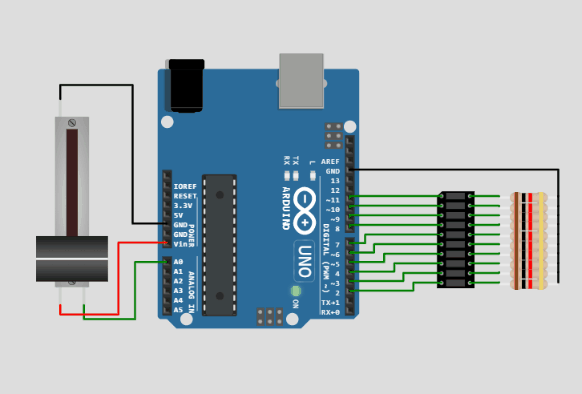Virtual Arduino Simulator - LED Bar graph

LED Bar project

In this project, LED bar is used to show the analog value read on the LED bar beautifully.

```        `// Your code here`
```Wokwi Arduino Simulator - LED Bar part simulation

## Code

```        ```/*
LED bar graph

https://wokwi.com/arduino/projects/309829489359061570

Turns on a series of LEDs based on the value of an analog sensor.
This is a simple way to make a bar graph display. Though this graph uses 10
LEDs, you can use any number by changing the LED count and the pins in the
array.

This method can be used to control any series of digital outputs that depends

The circuit:
- LEDs from pins 2 through 11 to ground

created 4 Sep 2010
by Tom Igoe

This example code is in the public domain.

https://www.arduino.cc/en/Tutorial/BuiltInExamples/BarGraph
*/

// these constants won't change:
const int analogPin = A0;   // the pin that the potentiometer is attached to
const int ledCount = 10;    // the number of LEDs in the bar graph

int ledPins[] = {
2, 3, 4, 5, 6, 7, 8, 9, 10, 11
};   // an array of pin numbers to which LEDs are attached

void setup() {
// loop over the pin array and set them all to output:
for (int thisLed = 0; thisLed < ledCount; thisLed++) {
pinMode(ledPins[thisLed], OUTPUT);
}
}

void loop() {
// map the result to a range from 0 to the number of LEDs:
int ledLevel = map(sensorReading, 0, 1023, 0, ledCount);

// loop over the LED array:
for (int thisLed = 0; thisLed < ledCount; thisLed++) {
// if the array element's index is less than ledLevel,
// turn the pin for this element on:
if (thisLed < ledLevel) {
digitalWrite(ledPins[thisLed], HIGH);
}
// turn off all pins higher than the ledLevel:
else {
digitalWrite(ledPins[thisLed], LOW);
}
}
}```
```

## Questions on Arduino Simulator?

The below article answers the following questions

• How to draw and connect using wires?
• How to add a part in the Wokwi Simulator?
• How to change wire colours?
• How to delete a part on Wokwi?
• How to move, rotate parts on the Wokwi Simulator and more

https://www.hackster.io/Hack-star-Arduino/how-to-use-wokwi-arduino-simulator-what-is-wokwi-304e6b

Support/feedback/suggestions?

you have many ways to ask for help, suggest a feature or share your feedback

Open an issue on GitHub